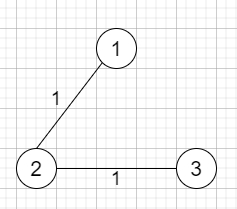# Optimize Water Distribution in a Village in Python

Suppose there are n houses in a village. We have to supply water for all the houses by building wells and laying pipes. For each house i, we can either build a well inside it, the building cost will be wells[i], or pipe in water from another well to it. The costs to lay pipes between houses are given by the array pipes, where each pipes[i] is [house1, house2, cost] represents the cost to connect house1 and house2 together using a pipe. These connections are bidirectional. We have to find the minimum total cost to supply water to all houses.

So, if the input is like n = 3, wells = [1,2,2], pipes = [[1,2,1],[2,3,1]], then the output will be 3as from the above image shows the costs of connecting houses using pipes. The best strategy will be to build a well in the first house with cost 1 and connect the other houses to it with cost 2 so the total cost is 3.

To solve this, we will follow these steps −

• Define a function find(). This will take a

• if parent[a] is same as -1, then

• return a

• parent[a] := find(parent[a])

• return parent[a]

• Define a function union(). This will take a,b

• parent_a := find(a)

• parent_b := find(b)

• if parent_a is same as parent_b, then

• return True

• parent[parent_b] := parent_a

• return False

• From the main method do the following −

• parent := make a list of size n + 1, fill this with -1

• counter := 0

• for i in range 0 to size of well, do

• insert [0, i+1, well[i]] at the end of pipes

• counter := counter + 1

• sort the pipes array based on the cost

• cost := 0

• for each i in pipes, do

• source := i

• destination := i

• temp := i

• if union(source,destination) is false, then

• cost := cost + temp

• return cost

Let us see the following implementation to get better understanding −

## Example

Live Demo

class Solution(object):
def find(self, a):
if self.parent[a] == -1:
return a
self.parent[a] = self.find(self.parent[a])
return self.parent[a]
def union(self,a,b):
parent_a = self.find(a)
parent_b = self.find(b)
if parent_a == parent_b:
return True
self.parent[parent_b] = parent_a
return False
def minCostToSupplyWater(self, n, well, pipes):
self.parent = [-1 for i in range(n+1)]
counter = 0
for i in range(len(well)):
pipes.append([0,i+1,well[i]])
counter+=1
pipes = sorted(pipes,key=lambda v:v)
cost = 0
for i in pipes:
#print(i)
source = i
destination = i
temp = i
if not self.union(source,destination):
cost+=temp
return cost

ob = Solution()
print(ob.minCostToSupplyWater(3, [1,2,2], [[1,2,1],[2,3,1]]))

## Input

3, [1,2,2], [[1,2,1],[2,3,1]]

## Output

1